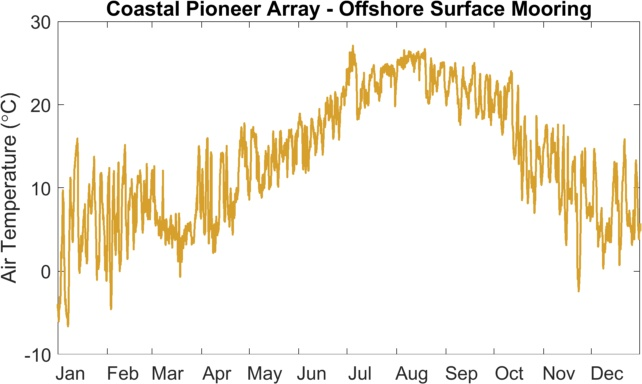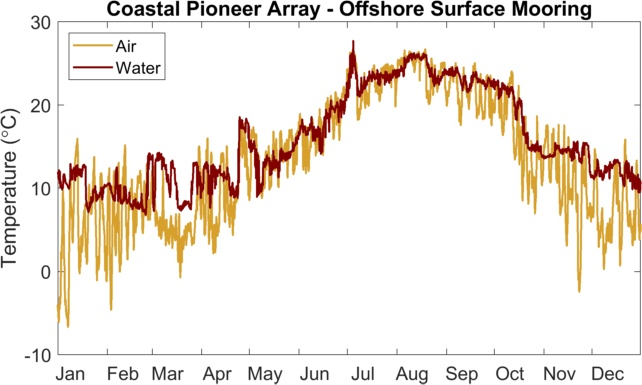## Lab 2.1 – Reading a time series graph

Fundamental concept: Apply graph reading skills to identify and understand variability in data and identify trends in a time series graph
Preparation for: Lab 4 – Volcanoes, Lab 5 – Density, Lab 6 – Waves, Lab 7 – Primary Production, Lab 8 – Anoxic Events
Estimated time to complete: 30 minutes
Materials needed (none)

Time series plots are often used to show daily, seasonal and interannual changes in some property.  You have probably experienced daily and seasonal changes in temperature.  There are daily and seasonal changes in temperature in the ocean too so fish, whales and other marine organisms feel them.  The water in the ocean has a moderating effect on temperature change though, so changes are not as extreme as those experienced on land.  For example, the lowest recorded temperature on land is -88°C (-126°F) and the highest is 58°C (136°F) .  Compare that to the temperature range in the ocean, -1°C (30.2°F) to 30°C (86°F).  Interestingly, the large temperature range on land seems to have resulted in land animals being better adapted to temperature changes.  As the oceans warm even a little bit scientists are finding that it affects both the number of organisms in an area and the number of species.

In this activity you will investigate time series from the Coastal Pioneer Array, near the edge of the U.S. east coast continental shelf.

### Orientation & Interpretation Questions:Figure 2.1.1. Air temperature in 2018 at the Coastal Pioneer array.

Figure 2.1.1 shows the air temperature as measured at the OOI Coastal Pioneer Offshore mooring over one full year. Let’s explore this graph and see if we can make sense of it.

Answer the following questions to become familiar with this time series plot (Figure 2.1.1).

1. What was the start and end date for data collection?
2. What variable is plotted on the y-axis of this graph and what are the units?
3. How does this variable vary throughout the year? Does the variation make sense based on your knowledge of seasonal temperature variation?
4. A trend in a time series is when there is a gradual change (i.e., an increase or decrease) over time, depth or distance. What trends do you see in these data?

The graph (Figure 2.1.2) below shows a small part of the data set you just examined.Figure 2.1.2. Air temperature in October 2018

1. What is the start and end date of the data collection in Figure 2.1.2?
2. Notice that there is a gap in the data. What does that gap mean?
3. Are there any trends in the data? What do you think causes them?
4. What are the maximum and minimum values in the data? Estimate to the best of your ability from the graph.
5. Considering your answers to these questions can you explain the “messiness” of the data in the first graph (Figure 2.1.1)?

Sometimes it is easier to look at long term data by taking averages, for example it is often useful to average temperature data over a 24 hour period to remove the temperature change that occurs between day and night. For other studies an average of all the data collected during a month may be appropriate. The graph (Figure 2.1.3) below shows the same data set examined in the previous graphs, but the data points are monthly averages of temperature.Figure 2.1.3. Monthly averaged air temperature in 2018

1. What are the maximum and minimum temperature values, and what do you think causes them?
2. The graph above (Figure 2.1.3) looks much “neater” than the previous graphs. This is because much of the detail has been lost in the averaging process. Is this a fair trade-off if you want to look at seasonal changes in temperature?
3. Is one or more trend(s) evident in the monthly mean temperature data shown in the figure 2.1.3? Describe the trend(s).

Sometimes we want to compare two data sets to see if they are related. One way to do that is to plot them on the same set of axes. Below is the same graph of air temperature but now the sea surface temperature has been added to it.Figure 2.1.4. Temperature measured in the air (yellow) and surface ocean water (red) in 2018

1. Based on Figure 2.1.4, are there any trends in the water temperature data? What are they?
2. What is the maximum and minimum in the water temperature and when do they occur (Figure 2.1.4)?
3. How does the seasonal range in water temperature compare to the seasonal range in air temperature? Why do you think that they aren’t the same?
4. Is water temperature correlated with air temperature? In other words, as one temperature series increases or decreases does the other one also change?

When scientists want to compare two different types of measurements they can plot them on the same graph, but have to use different y-axes. Examine the graph below (Figure 2.1.5), which shows the surface water temperature data and surface salinity data. Note that the temperature data are plotted relative to the y-axis on the LEFT, while the salinity data are plotted relative to the y-axis on the RIGHT. The units given for salinity are PSU. This stands for practical salinity units.

Figure 2.1.5. Temperature (red) and salinity (blue) of the surface ocean water in 2018.

1. What are the maximum and minimum values for sea surface salinity in Figure 2.1.5?
2. Are there any trends in the salinity data? What are they?
3. Notice that you can click and drag the two scroll bars at the bottom of the graph to zoom in and out to different portions of this graph. Zoom in so that only April and May are visible. Does there appear to be a correlation between sea surface temperature and salinity? If so, what type of relationship did you find between temperature and salinity?
4. Now zoom out to view the rest of the year. Does the same relationship between sea surface salinity and temperature hold for the whole year?

#### Reflection Question:

1. Look back to the beginning of this exercise to see the ranges in temperature in the ocean versus on land.  Now examine the Coastal Pioneer Array data in Figure 2.1.4.  What is the measured range for air and ocean temperatures in the Coastal Pioneer Array data?  How does this compare to the ranges for air and ocean temperature extremes?